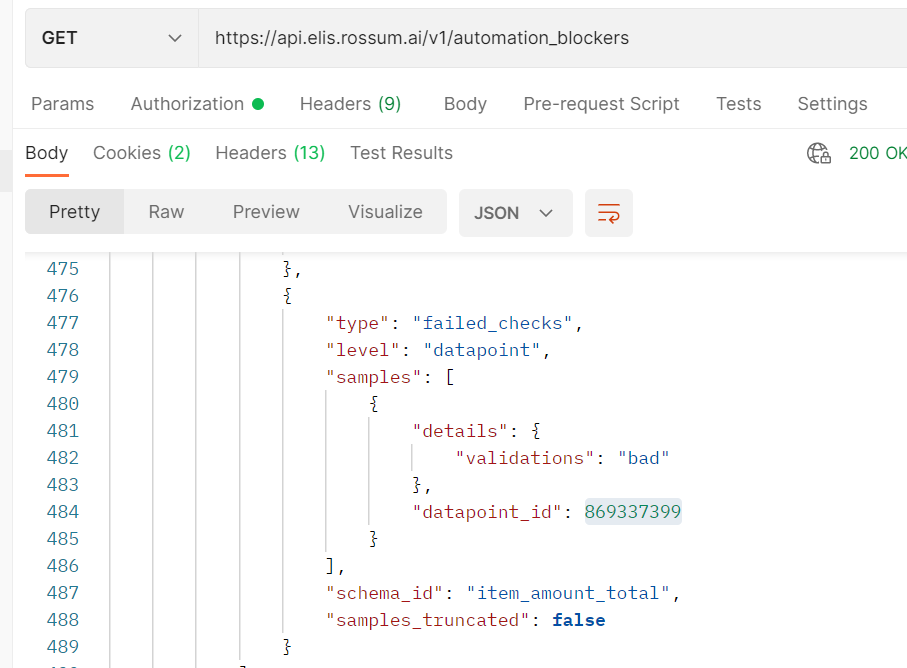# Built-in checks overview

The first step of Rossum's automation pipeline is about Data Integrity. That means the relationships between fields on the document can be described by formal rules.

In general, each field can be checked against multiple rules because it can be in relationship with various fields on the document. Therefore, evaluating all of the rules for a given field can result into a positive or negative check that either automates the field or blocks the automation.

At Rossum, we have been tracking general Data Integrity rules for quite some time and they became part of the Automation decision process. Such rules (described in sections below) are applied by default to all accounts.

As we are trying to be as transparent as possible, we have provided the list of rules as well as the effects it has on the Automation. Please let us know at [email protected] if you are missing some rules that are common in Accounts Payable domain.

# Positive vs. Negative checks

We distinguish the built-in checks into positive and negative checks. If we would run an extracted value through all the rules, and at least one rule would be satisfied then the field would be automated. We call this a positive check of a field.

In contrast, if we would evaluate all the rules for a specific field and all the rules would be evaluated as "False" then the automation of such field will be blocked even if the confidence score of such field is over the threshold. This is called a negative check.

Any of extracted values can result into negative check and thus would stop automation of the document. Additionally, not all the rules can result in positive checks and such rules have a note "can be used only as part of a negative check".

# Data Integrity in Accounts Payable domain

Accounts Payable domain tends to have quite a high Data Integrity level of the captured data. Therefore, we have prepared rules that the AI considers when deciding about automating an Accounts Payable document.

# Variables forming the Data Integrity rules

The rules we consider are based on the Rossum's AI Engine outputs (rir_field_names) mapped to your fields defined in the Extraction schema.

Let's explain how it works on the example below:

``````{
"category": "datapoint",
"constraints": {
"required": false
},
"default_value": null,
"format": "D/M/YYYY",
"id": "issue_date",
"label": "Issue date",
"rir_field_names": [
"date_issue"
],
"type": "date"
},
{
"category": "datapoint",
"constraints": {
"required": false
},
"default_value": null,
"format": "D/M/YYYY",
"id": "due_date",
"label": "Due date",
"rir_field_names": [
"date_due"
],
"type": "date"
}
``````

The above defined "issue_date" field is mapped to the AI's output "date_issue". Once Rossum sees that a fields value was filled with "date_issue" AI output, Rossum checks whether the "due_date" field, filled with "date_due" rir_field name, complies with the formula:

``````0 <= (date_due - date_issue).days <= 120
``````

# Accounts Payable rules

Below is the list of Accounts Payable automation rules, divided into categories. As mentioned above, the rules are based on the Rossum's AI Engine outputs.

# Amounts

The Amounts category works on top of the AI Engine Amounts outputs. All the rules are used for both positive and negative checks.

Rules:

1. amount_total_base + amount_total_tax == amount_total (including rounding if available)
2. amount_paid + amount_due == amount_total (including rounding if available)

# Dates

The Dates category works on top of the AI Engine Dates outputs.

Rules:

1. 0 <= (date_due - date_issue).days <= 120 (can be used only as part of a negative check)
2. 0 <= (date_issue - date_uzp).days < 90 (can be used only as part of a negative check)
3. (date_due - date_issue).days == terms.days

# Tax details

The Tax details category works on top of the AI Engine Tax details outputs. All the rules are used for both positive and negative checks.

Rules:

1. tax_detail_base * tax_detail_rate == tax_detail_tax
2. tax_detail_tax + tax_detail_base == tax_detail_total

# Line items

The Line items category works on top of the AI Engine Line items outputs. All the rules are used for both positive and negative checks.

Rules:

1. table_column_amount_base * table_column_rate == table_column_tax
2. table_column_amount == table_column_amount_base + table_column_tax
3. table_column_amount_total_base == table_column_amount_base * table_column_quantity
4. table_column_amount_total == table_column_amount * table_column_quantity
5. table_column_description is not Null (This rule is not applied by default. Contact us at [email protected] if you would like this rule to be applied.)

# Amounts vs. Tax details

The Amounts category can be cross checked with the Tax details category. The checks are built on top of Amounts outputs and Tax details outputs. All the rules are used for both positive and negative checks.

Rules:

1. amount_total == SUM(tax_detail_total) (including rounding if available)
2. amount_total_tax == SUM(tax_detail_tax) (including rounding if available)
3. amount_total_base == SUM(tax_detail_base) (including rounding if available)

# Amounts vs. Line items

The Amounts category can be cross checked with the Line items category. The checks are built on top of Amounts outputs and Line item outputs. All the rules are used for both positive and negative checks.

Rules:

1. amount_total == SUM(table_column_amount_total) (including rounding if available)
2. amount_total_base == SUM(table_column_amount_total_base) (including rounding if available)
3. amount_total_tax == SUM(table_column_tax) (including rounding if available)

# How does this help to automate the documents?

Once knowing that some field's rules can be evaluated with a positive or a negative check, we will update the validation sources of the datapoint accordingly. Example of a positive check:

``````validation_sources:["checks"]
``````

Or the validation source will be empty for a field which denotes a negative check:

``````validation_sources:[]
``````

Additionally, you can see that the negative check was applied by getting the automation_blocker field on the annotation object.### 📘Combining built-in checks with other automation components

Learn how the different automation components interact together to automate the documents as well as how the automation works with hidden and required fields.